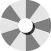### My Cart

Close

F r e e   S h i p p i n g   O n   A l l   O r d e r s   O v e r      \$ 1 2 0                F o r   A   L i m i t e d   T i m e   O n l y                    U p   T o   50%   O f f   O n   A c t i v e w e a r

F r e e   S h i p p i n g   O n   A l l   O r d e r s   O v e r      \$ 1 2 0                F o r   A   L i m i t e d   T i m e   O n l y                    U p   T o   50%   O f f   O n   A c t i v e w e a r

F r e e   S h i p p i n g   O n   A l l   O r d e r s   O v e r      \$ 1 2 0                F o r   A   L i m i t e d   T i m e   O n l y                    U p   T o   50%   O f f   O n   A c t i v e w e a r

# Blouses

Spin to win